• Post Author:

##### Non-electrolytes

The substances which do not conduct electricity in the molten state or in aqueous solution are called non-electrolytes. Non-polar organic compounds are non-electrolytes. For example: sugar, urea, etc.

##### Electrolytes

The substances which conduct electricity in the molten state or in aqueous solution are called electrolytes. Ionic compounds and highly polar organic ( covalent) compounds are electrolytes. For example: HCl, NaCl, NaOH, H2SO4, CH3COOH, etc.

Electrolytes are further classified as:

Strong electrolytes : The electrolytes which are almost completely ionized in aqueous solution are called strong electrolytes. For example: HCl, H2SO4, HNO3, NaOH, KOH, NaCl, Na2SO4, etc.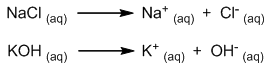Weak electrolytes : The electrolytes which ionize to small extent in aqueous solution are called weak electrolytes. For example: CH3COOH, H2CO3, NH4OH, H3PO4, HCN, etc.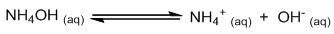##### Ionic equilibrium

The weak electrolytes are ionized only partially (feebly). The ions produced as a result of ionization of weak electrolytes are present in dynamic equilibrium with the unionized molecules. Such equilibrium is called ionic equilibrium.

Thus, the ionic equilibrium is the equilibrium which is established between the unionized molecules and the ions in solution of weak electrolytes.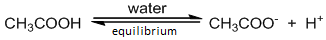##### Degree of ionization (dissociation)

The fraction of the total number of molecules of an electrolyte which ionizes into ions is called degree of ionization or degree of dissociation. It is denoted by α .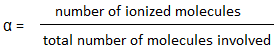Factors affecting degree of ionization :

• Concentration : Degree of ionization is inversely proportional to the concentration of solution.
• Nature of electrolyte: strong electrolytes are totally ionized in their solution, hence degree of ionization is 1. But weak electrolytes are partially ionized and degree of ionization is always very less than 1.
• Dilution or volume : Degree of ionization is directly proportional to the dilution (volume) of solution.
• Temperature : Degree of ionization of weak electrolytes increases with increase in temperature.

#### Ostwald’s dilution law

Statement : At constant temperature, degree of ionization of a weak electrolyte is directly proportional to the square root of dilution(volume) and inversely proportional to the square root of concentration.

Mathematically ;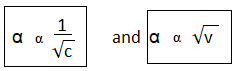Derivation : Let us consider the ionization of weak electrolyte AB in water. Suppose C mole-1 be the concentration of the electrolyte and α be the degree of ionization. Then,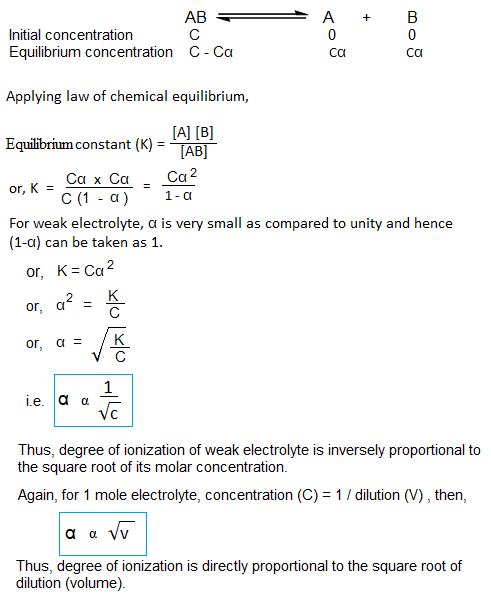From Ostwald’s dilution law,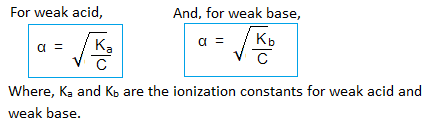Limitation of Ostwald’s dilution law : This law is applicable only to weak electrolytes and it fails in case of strong electrolyte.

#### Different Concepts of Acids and Bases

1. Arrhenius Concept of Acids and Bases :

An acid is a compound which gives H+ ions in water. Eg.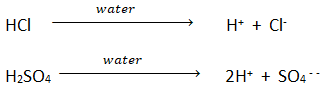A base is a compound which gives OH ions in water. Eg.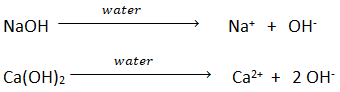Limitations of Arrhenius concept :

• Arrhenius concept is limited to aqueous medium only. It fails to explain the behavior of acids and bases in non-aqueous medium.
• It fails to explain the acidic nature of CO2, SO2, NO2 etc. which do not contain any hydrogen.
• It fails to explain the basic nature of CaO, NH3, Na2CO3, etc. which do not contain hydroxyl group.
• CH4 contains hydrogen but is not an acid. Similarly, CH3OH have OH group but is not a base.

2. Bronsted – Lowry Concept of acids and bases :

An acid is a substance that can donates a proton.

A base is a substance that accepts a proton. Eg.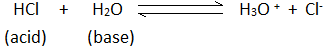Here HCl donates proton and H2O accepts proton. Hence HCl is an acid and H2O is base.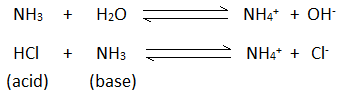Limitations of Bronsted – Lowry Concept :

• Substances like BF3, AlCl3, etc. do not contain any hydrogen and hence cannot give a proton but behave as acids.
• It can not explain the reaction between acidic oxide like CO2, SO2, SO3, etc and the basic oxides like CaO, MgO, etc. where is no proton transfer.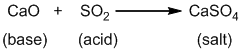3. Lewis concept of acids and bases:

• An acid is a substance which can accept a pair of electrons.
• A base is a substance which can donate a pair of electrons.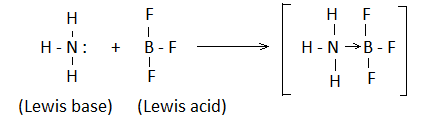Limitations of Lewis concept:

• It does not explain the acidic behavior of well known protic acids such as HCl, H2SO4, etc. which do not form coordinate bond with bases.
• It does not explain the relative strength of acids and bases , as it is not based on ionization.

##### Conjugate Acid- Base pair

A pair of acid and base that differs from each other by a single proton(H+) is known as conjugate acid – base pair.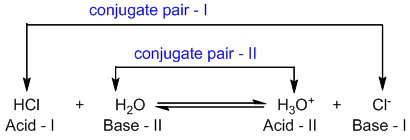In this reaction Hydrochloric acid and chloride ion are conjugate acid- base pair. Similarly, hydronium ion (H3O+) and water are another conjugate acid – base pair.

Note: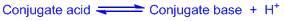Some examples of Acids and Bases :

Strong acids : HI > H2SO4 > HCl > HNO3 , etc.

Strong bases : NaOH > KOH > Mg(OH)2 > Ca(OH)2 , etc.

Weak acids : HCOOH, CH3COOH, HF, H2S, H2CO3, etc.

Weak bases : NH3, NH4OH, etc.

#### Salt and it’s types

A salt is an ionic compound that results from the neutralization reaction of an acid and a base.

Eg. NaCl, KCl, Na2CO3, CuSO4 etc.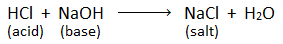Arrhenius concept: Those substances which gives cation except H+ and anion except OH when dissolves in water is Arrhenius salt. Eg. NaCl.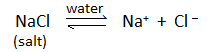1. Acidic salt : Salt formed by the reaction between strong acid and weak base is called acidic salt.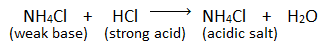2. Basic salt : Salt formed by the reaction between strong base and weak acid is called basic salt.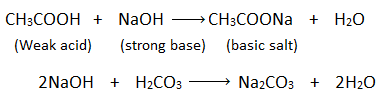3. Neutral salt : Salt formed by the reaction between strong acid and strong base is called neutral salt.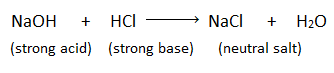#### Ionic product of water, PH and POH

Pure water is a weak electrolyte and ionizes slightly as :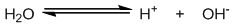Now, at equilibrium applying law of mass action,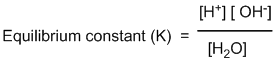Since, degree of ionization of weak electrolyte is very small, the concentration of unionized water can be taken as constant. Thus we can write,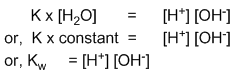Where, Kw is a constant and is known as ionic product of water. Its value is constant at particular temperature and varies with the change in temperature.

The value of ionic product of water (Kw) at 250C (i.e.298K) is found to be 10-14.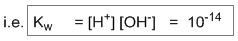Therefore, for pure water, [H+] = [OH] = 10-7 molL-1 (M)

Note: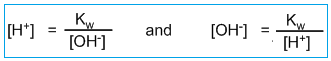##### PH and POH

pH of a solution is defined as the negative logarithm of hydrogen ion concentration.

i.e. pH = -log[H+]

pOH of a solution is defined as the negative logarithm of hydroxide ion concentration.

i.e. pOH = -log[OH]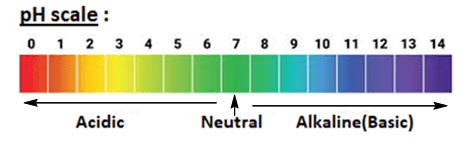##### Relation between PH and POH

We know that,

pH = -log[H+] and

pOH = -log[OH]

At 250C, ionic product of water(Kw) = [H+][OH] = 10-14

Or, [H+][OH] = 10-14

Taking log on both sides, we get,

log[H+] + log[OH] = -14log10

or, -log[H+] + {-log[OH]} = 14

Hence, pH + pOH = 14

#### Hydrolysis of salt

Acid reacts with base to give salt and water. Some salts when dissolved in water interact with water and break down into parent acids and bases. such reaction is known as salt hydrolysis. For example:

When ammonium chloride is dissolved in water gives hydrochloric acid (i.e strong acid) and ammonium hydroxide (i.e weak base).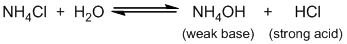Strong acid ionizes completely in the solution where as weak base ionizes partially. Hence, the resulting solution is acidic as it contains excess of hydrogen ions.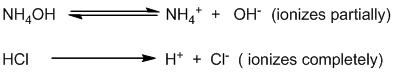Thus, the aqueous solution of ammonium chloride is acidic in nature.

Q) Explain why the aqueous solution of MgSO4 is acidic in nature?

Q) Washing soda solution is alkaline. Why?

Note :

The acidic salt (salt of a strong acid with a weak base) gives acidic solution when dissolved in water.

The basic salt (salt of a strong base with a weak acid) gives basic solution when dissolved in water.

The neutral salt (salt of a strong acid with a strong base) gives neutral solution when dissolved in water.

#### Solubility product

“Solubility product is defined as the product of ionic concentration in saturated solution at constant temperature.”

If a pinch of sparingly soluble salts like AgCl, BaSO4, PbCl2, etc. is dissolved in water, a saturated solution will be formed in which there exists an equilibrium between solid salt and its ions.

For example,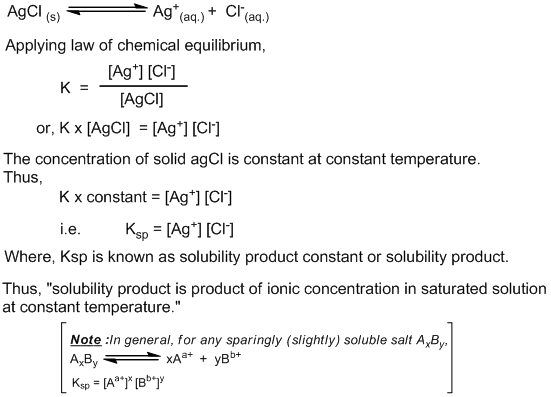Relation between solubility and solubility product:

Let, solubility of sparingly (weakly) soluble salt is S mole L-1 , then,

1. For AB type salts :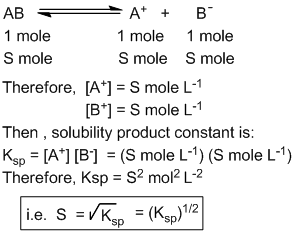1. For AB2 type salt :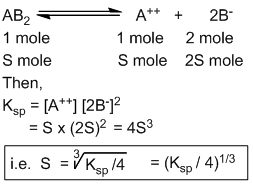2. For AB3 type salt :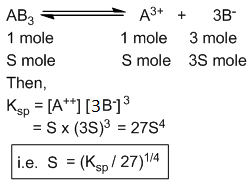3. For A2B3 type salt: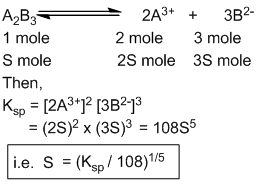Difference between solubility product and Ionic product :

Both ionic product and solubility product represent the product of the concentrations of the ions in the solution. The term ionic product has a broad meaning since, it is applicable to all types of solutions, either unsaturated or saturated or supersaturated.

On the other hand, the term solubility product is applied only to a saturated solution in which there exists a dynamic equilibrium between the undissolved salt and the ions present in solution. Thus, the solubility product is in fact the ionic product for a saturated solution at a constant temperature.

Relation between ionic product and solubility product and precipitation:

A sparingly soluble salt precipitates from a solution only when its ionic product exceeds solubility product value. Thus when

Ionic product > Ksp, solution is supersaturated and hence precipitation takes place.

Ionic product < Ksp, solution is unsaturated and hence precipitation does not take place.

Ionic product = Ksp, solution is saturated.

#### Common ion effect

When a strong electrolyte having one ion common to weak electrolyte is added to the solution of weak electrolyte then the ionization of weak electrolyte is decreased. This effect is called common ion effect.

For example:

a. Ionization of ammonium hydroxide is decreased by the addition of ammonium chloride.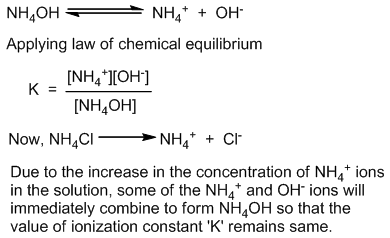b. Ionization of acetic acid is decreased by the addition of sodium acetate.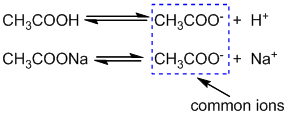#### Application of common ion effect and solubility product principle in precipitation reaction:

Precipitation of metal ions in qualitative analysis (salt analysis):

In qualitative analysis, metal ions are precipitated as their insoluble salts.

a. Precipitation of sulphides of group-II metal ions:

In group II of qualitative analysis, when H2S gas is passed into the original solution containing HCl then metal ions such as Pb++, Cu++, Hg++, Cd++,etc. are precipitated in the form of their sulphides. The ionization of H2S is suppressed due to the presence of common hydrogen ion(H+) produced by HCl.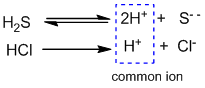Here, the concentration of S– – ions is decreased due to common ion effect. The solubility product of sulphides of group II metal ions is relatively low. Therefore, the ionic product of sulphide ions and metal ions exceeds the solubility product and hence the the metal ions of group II are precipitated as sulphide (PbS, CuS, HgS, CdS, etc.)

But, the reduced concentration of S– – ions is not sufficient to precipitate metal ions of other groups (III, IV and V) because their solubility products are comparatively high.

b. Precipitation of hydroxides of group-IIIA metal ions:

In group IIIA of qualitative analysis, when NH4OH is passed into the original solution containing NH4Cl then metal ions such as Fe+++, Al+++, Cr+++,etc. are precipitated in the form of their hydroxides. The ionization of NH4OH is suppressed due to the presence of common NH4+ ion produced by NH4Cl.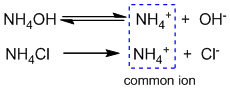Here, the ionic product of hydroxide ions and metal ions exceeds the solubility product of metal hydroxides of group IIIA only and hence  the metal ions of group IIIA are precipitated as their hydroxides {Al(OH)3, Fe(OH)3, Cr(OH)3, etc.}

But, the reduced concentration of OH ions is not sufficient to precipitate metal ions of other groups ( IV and V) because their solubility products are comparatively high.

Note: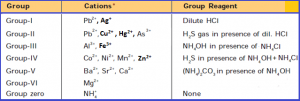#### Buffer solution

A solution which can resist change in pH even on addition of small amount of acid or base is called buffer solution.

Example: In a biological system, blood is an example of buffer and its pH remains almost constant to 7.4.

Buffer solutions are of two types:

i. Acidic buffer: The buffer prepared by mixing equimolar quantities of weak acid and its salt with strong base is known as acidic buffer. The pH value of acidic buffer is less than 7.

Example: A solution containing equimolar quantities of acetic acid (CH3COOH) and sodium acetate (CH3COONa) is acidic buffer.

ii. Basic buffer: The buffer prepared by mixing equimolar quantities of weak base and its salt with strong acid is known as basic buffer. The pH value of basic buffer is more than 7.

Example: A solution containing equimolar quantities of ammonium hydroxide (NH4OH) and ammonium chloride (NH4Cl) is acidic buffer.

#### Numerical Problems

Calculation of pH and pOH:

Strong acid and strong base:

1. Calculate H+, OH ion concentration and pH at 250C.
2. 0.001M HCl solution.
3. 0.05M H2SO4 solution.
4. 0.04M NaOH solution.
5. 0.020M Ba(OH)2.
6. 0.05N H2SO4 solution.
7. Calculate the [H+], [OH] and pH in a solution in which 2g NaOH is dissolved in a 2 litre solution.
8. The pH of a solution is 6. Its hydrogen ion concentration is increased to 1000 times. What is the pH of the resulting solution?
9. What is the new pH if 100ml of a solution of HCl with pH=0.52 is diluted by the addition of 200ml of pure water?
10. The pH of sulphuric acid solution is 4. What is the molarity of the solution?
11. The pH of sulphuric acid solution is 3. What is the normality of the solution?

Very dilute (aq.) solution:

1. Calculate pH of 1.0×10-8M HCl solution.
2. Calculate pH of an aqueous solution containing 10-7 moles NaOH per litre.
3. Sodium hydroxide having pH=8 is diluted 1000 times. Calculate the pH of the diluted base.

pH of Weak acid and base:

1. Calculate pH of 0.2M solution of hydrocyanic acid (HCN). Given, ionization constant of HCN=7.2×10-10
2. If the pH of 0.1M solution of a weak acid is 3. Calculate the value of Ka for the acid.

pH of mixture:

1. 100 ml of 0.1N HCl is mixed with 300ml of 0.05N HNO3. Calculate the pH of the resulting mixture.
2. 10cc of N/2 hydrochloric acid, 30cc of N/10 nitric acid and 60cc of N/5 sulphuric acid are mixed together. Calculate pH of the mixture.
3. 1ml of 0.1N HCl is added to 999ml of NaCl. Calculate the pH of the resulting solution.
4. Calculate pH of a mixture obtained by mixing 75ml of 0.2M HCl and 25ml of 0.2M NaOH solution.
5. Equal volume of two solutions having pH=5 and pH=10 are mixed. Find the pH of resulting mixture.
6. Calculate pH of the solution obtained by mixing equal volume of solutions with pH=4 and pH=5.
7. Calculate pH of solution obtained by mixing 50cc solution of pH=1 and 100cc solution of pH=2.
8. Equal volume of two solutions having pH=4 and pH=9 are mixed. Find the pH of the resulting mixture.
9. The pH of the resulting solution obtained by mixing 100ml of xM NaOH and 100ml of 0.1M H2SO4 is 1.699. Find the value of x.
10. 85 ml of 0.1 M H2SO4 solution is titrated with 0.1 N KOH solution. Find the pH of the solution when the acid is one-fourth neutralized.

Solubility and Solubility product constant relation:

1. The solubility of AgCl in water at 250C is found to be 1.87×10-3 gmL-1 . Calculate the solubility product of AgCl at this temperature. ( Ag = 107.9, Cl= 35.5)
2. If the solubility of PbI2 is 1.68×10-3 mol per liter, considering 90% ionization find it’s solubility product.
3. The solubility of CaF2 in water at 298 K is 1.7×10-3 gm per 100 cm3. Calculate the solubility product of CaF2 at given temperature.
4. The solubility product constant of BaSO4 in water at 250C is 1×10-10 mol2L-2. Calculate the solubility of BaSO4 in gm per liter. ( Ba = 137 )
5. 0.00143 gm of AgCl dissolved in 1 liter of water at 250C to form a saturated solution. What is the solubility product of the salt?
6. Calculate minimum volume of water required to dissolve one gram of CaSO4 at 298 K. (Ksp= 9.1×10-6)

Common ion Effect:

1. The Solubility product of CaCO3 is 8.7×10-9 at 250C. Calculate its solubility in 0.1M CaCl2.
2. The solubility product of Ferric hydroxide is 1.7×10-18 at a given temperature. Find its solubility in 0.1 M NaOH at same temperature.
3. The solubility of AgCl in water at 298 K is 1.43×10-3 gmL-1. Calculate its solubility in 0.5 M KCl solution. Also, calculate the amount of AgCl precipitated.
4. Calculate solubility of AgCl(s) in (a) pure water (b) a solution of 0.1M NaCl at 250C. [Ksp of AgCl = 1.0×10-10]
5. The solubility product of Ca(OH)2 at 298 K is 4.42×10-5. A 500 ml of saturated solution of Ca(OH)2 is mixed with an equal volume of 0.4 M of NaOH. What mass of Ca(OH)2 is precipitated?

Solubility product principle:

1. Given that the solubility product of BaSO4 is 1×10-10. Will a precipitate form when the equal volume of 2×10-8 M BaCl2 solution and 2×10-3 M Na2SO4 solution are mixed?